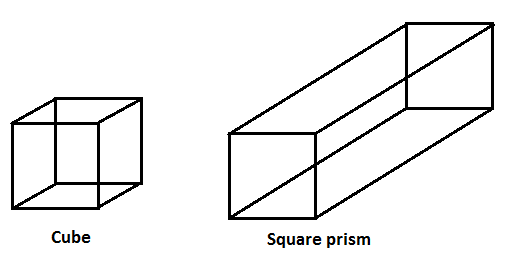QUESTION

# Is a square prism the same as a cube? Explain.

Hint: Start by defining a prism. Then write the definition of a square prism and a cube. Draw a diagram for both the figures. From the diagram, notice that a square prism is the same as a cuboid. Using this, we can say that all cubes are square prisms but not all square prisms are cubes. This is the final answer.

In this question, we need to find whether a square prism is the same as a cube or not.
Let us first define a prism.
A prism is simply two bases that are the same, joined together by flat sides to form an enclosed three-dimensional shape.
Let us define a square prism and a cube.
A square prism is a three-dimensional shaped cuboid figure whose bases are squares. The opposite sides and angles are congruent to each other.
In Geometry, a Cube is a solid three-dimensional figure, which has 6 square faces, 8 vertices and 12 edges. It is also said to be a regular hexahedron.
Shown below are figures of a square prism and a cube.As we can see from the figure above that a square prism is the same as a cuboid.
We know that a cube is a special type of cuboid (square prism) where the lengths in all three dimensions are the same.
In short, all cubes are square prisms but not all square prisms are cubes.
In order to be a square prism, it must have two square bases and a square cross section all the way through, but the third dimension does not have to be the same as the other two.

Note: In this question, it is very important to understand the definitions of a square prism and a cube and then identify the difference between the two. It is also important to realise that a square prism is the same as a cuboid.